### Home > CALC > Chapter 1 > Lesson 1.1.1 > Problem1-4

1-4.
1.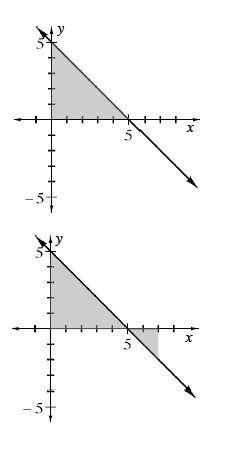Examine the graph the function f(x) = 5 − x at right. Homework Help ✎

1. Find the area of the shaded region using geometry.

2. Notice that the line dips below the x-axis when x > 5. We will consider the area below the x -axis as negative. Find A(f, 0 ≤ x ≤ 7).

3. Find k if A(f, 0 ≤ xk) = 0. Show how you obtained your solution clearly and completely.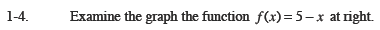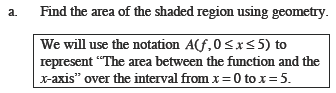Notice the familiar geometric shape.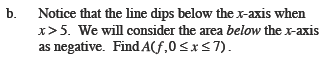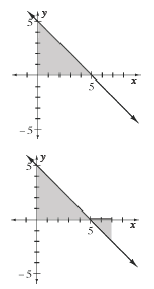Find the area of the negative region and combine
it with the positive area found in part (a).

A (f, 0 ≤ x ≤ 5) + A (f, 5 ≤ x ≤7)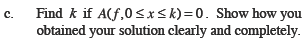A (f, 0 ≤ x ≤ 5) + A (f, 5 ≤ xk)

$[\text{area from part a}]+\frac{1}{2}(k-5)(-k+5)=\underline{ \ \ \ \ \ \ \ \ \ \ \ \ \ \ \ \ \ \ \ \ \ \ \ \ \ \ \ \ }$